## Maths KS1 & KS2

Maths is taught using the Kent Scheme as a basis. However, we use Big Maths at the start of most Maths lessons to help children learn about number and practice skills.

Fast paced!  Fun!  Big Maths is used extensively in Ashford Oaks to introduce

child-friendly terms such as ‘Switchers’ and ‘Learn-its’, to help them use numbers to

make them more confident and successful. It has a strong emphasis on mental maths

using number facts and times tables.

Some components of Big Maths: CLIC (Counting, Learn Its, Its nothing new and Calculation); Learn-its (Addition facts and times table facts, 72 facts in all); Beat That (Total Recall: a weekly timed test on their Learn Its).

The year groups are set respective targets to help guide our children towards the goal of maths competency.

Our Maths Targets

Year One Expectation: Squiggleworth Target

• know my finger doubles

• I can add numbers of objects to 10

• I can add or subtract 1

• I can order three numbers between 1 and 10

• I can write any given number from 1 –10

• I can solve an addition number sentence

• I can halve an even number of objects

• I can count up to 10 objects

• I know the missing number to make 10

• I can take away numbers of objects to 10

Year Two Expectation: Pim Target

• I can partition a 2- digit number

• I can spot odd  and even numbers

• I can solve repeated addition

• I know the fact families for 1-digit add 1-digit facts

• I know my doubles facts

• I can solve any 2-digit add 1-digit

• I can take any 1-digit number from any 2-digit number

• I can add 10s (multiples of 10)

• I know the missing number to make the next 10

• I can use my doubles facts to find halving facts

Year Three and Four Expectation: Mully Target

• I can partition a 3-digit number

• I can combine two or more tables facts  to solve division

• I can multiply multiples of 10 (2,3,4 & 5x only)

• When given a single fact I know the fact family

• I can partition a number with one decimal point

• I can solve any 3-digit add 3-digit

• I can solve any 3-digit take 3digit

• I can multiply and divide whole numbers by 10

• I know the missing number to make 100

• I can multiply a 2-digit by a 1-digit number

Year Five and Six Expectation: Count Fourways Target

• I can partition a number with three decimal points

• I know what multiples, factors and square numbers are

• I can combine 2 or more tables facts to solve division

• I can write fractions as decimals and percentages

• I can solve 2 digit x 2 digit

• I can solve any additions with 2 decimal points

• I can subtract numbers with hundredths

• I can multiply/divide whole numbers by 10 and 100

• I know the missing number to make 1000

• I can multiply tenths

Year Six Expectation: Pom Target

• I can combine 2 or more tables facts to solve decimal division

• I can order numbers with different decimal places

• I can combine 2 or more coin facts to solve division

• I can write fractions as decimals and percentages

• I can solve 3 digit x 2 digit

• I can solve any 2 decimal points + 1 decimal point number

• I can subtract numbers with different decimal places

• I can multiply/divide whole numbers and decimals by 10, 100

and 1000

• I know the missing decimal piece

• I can multiply hundredths

## EARLY YEARS FOUNDATION STAGE

The curriculum that we teach in Nursery and Reception classes meet the requirements set out in the National Curriculum at Foundation Stage. Our curriculum planning focused on the seven areas of learning: Personal, Social and Emotional development, Physical development, Communication and language, Literacy (RWI), Mathematical, Understanding of the world and Exploring and using media and materials.

Our school fully support the principle that young children learn through play and by engaging in well-planned structures activities.

At the end of the year we report on the Characteristics of Effective Learning which are: Playing and exploring, Active learning and Creating and Thinking Critically.

We also go on a number of school trips,  to different places linked to our foundation subjects.

Yearly we have some special trips. Every class is named after a village around Ashford and they visit their village on Village Day. Year 4 have the exciting opportunity to go camping for three days in May. Finally Year 6 go on a week-long Residential trip at the start of the year.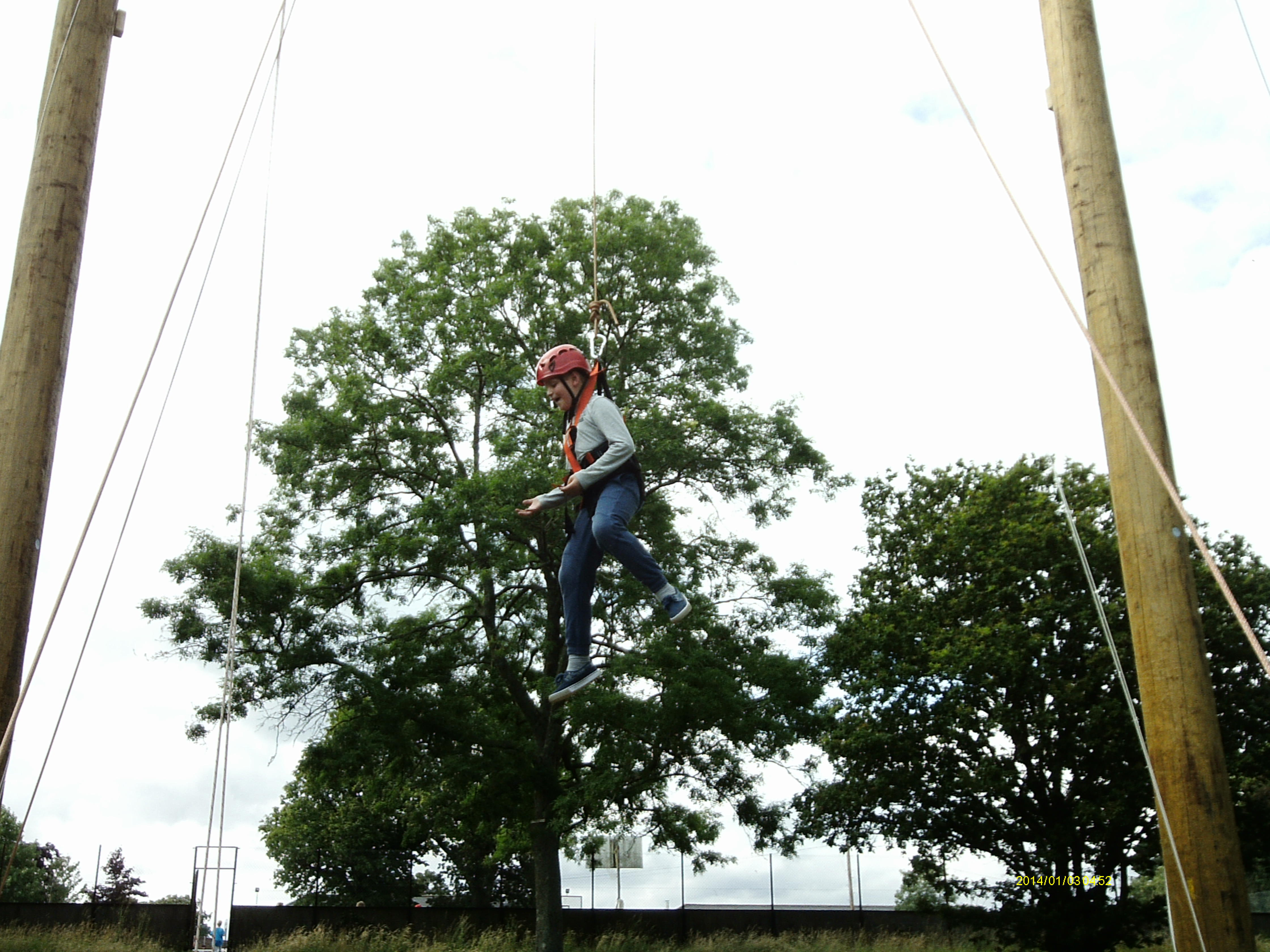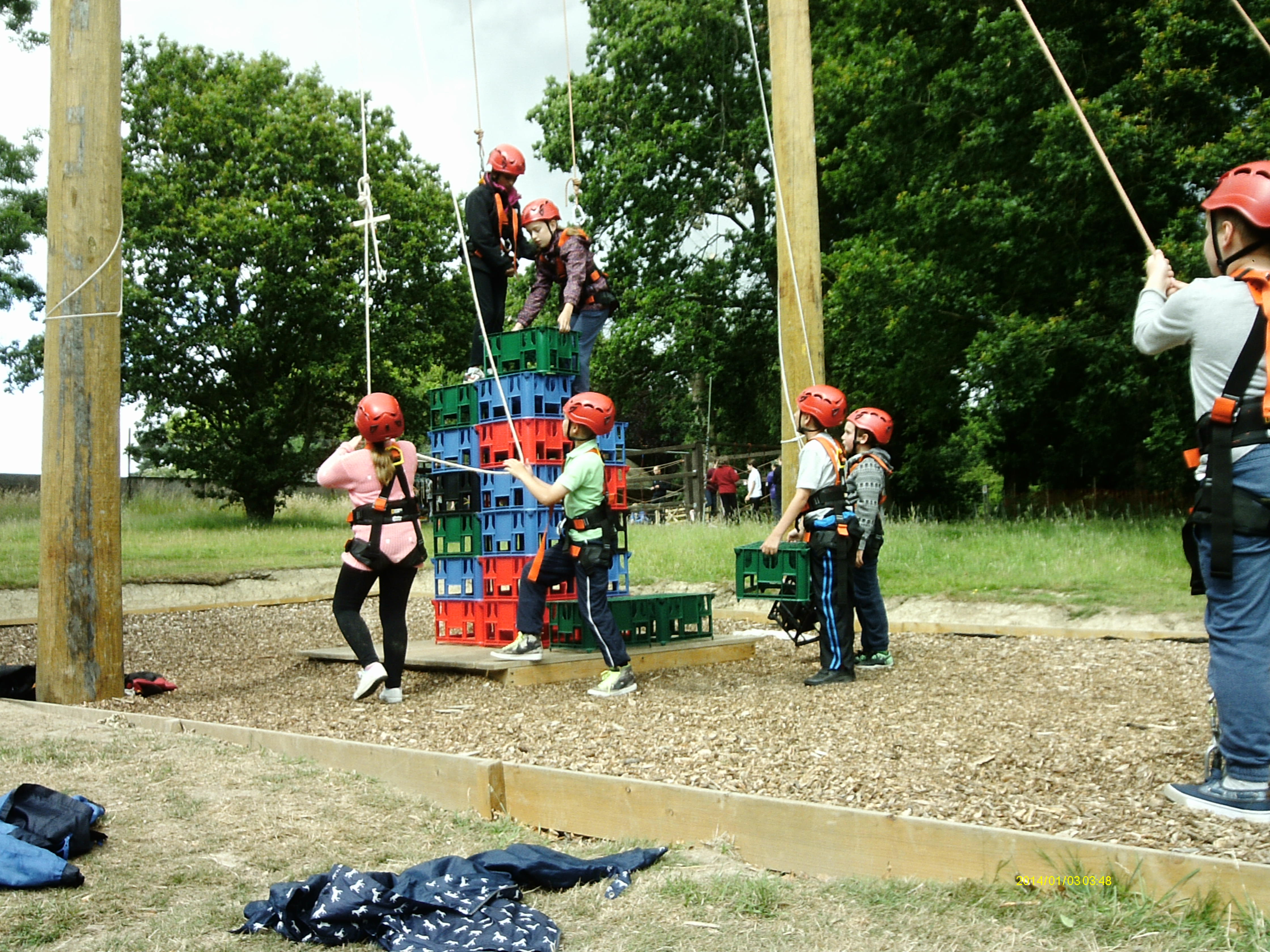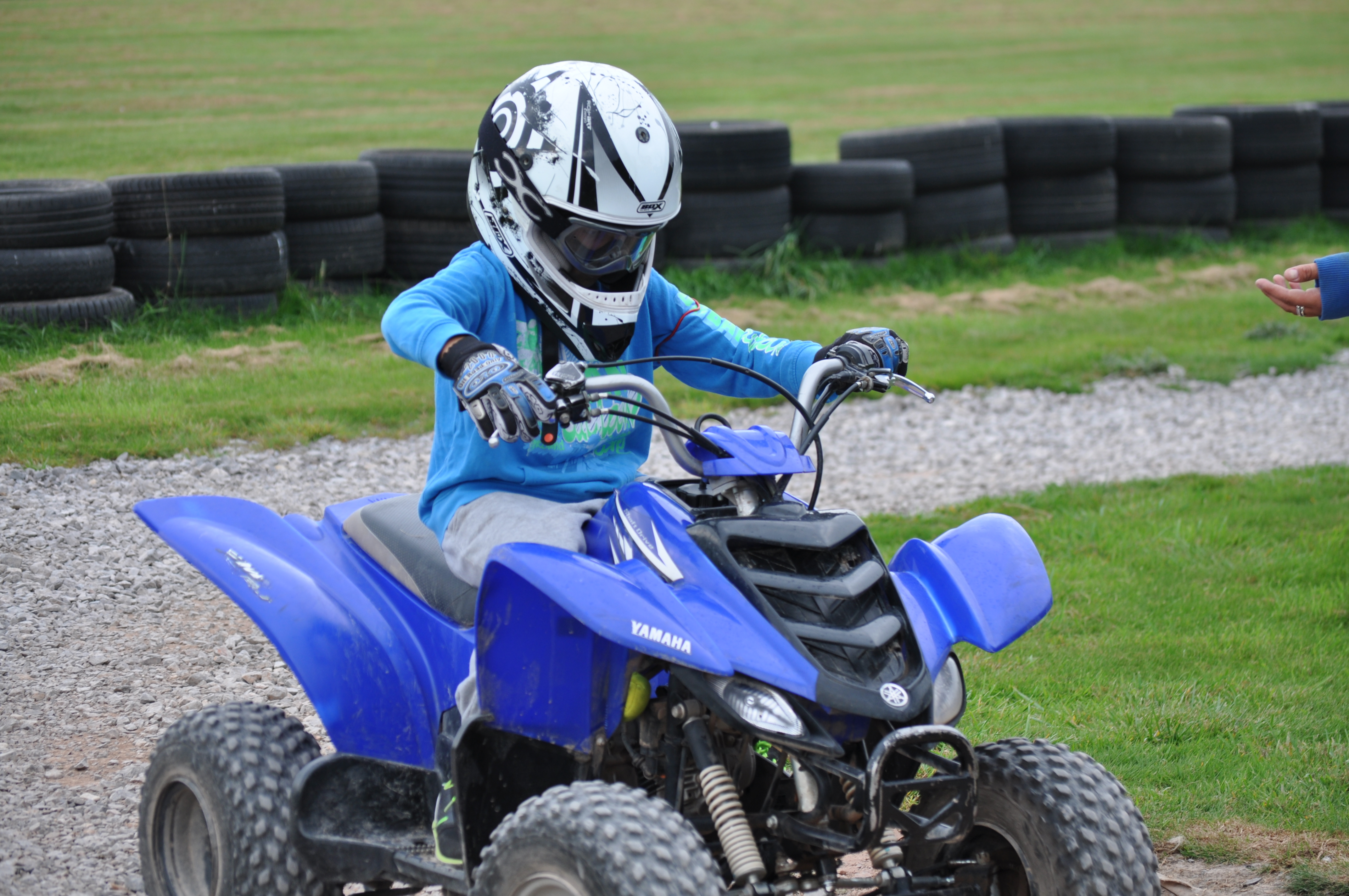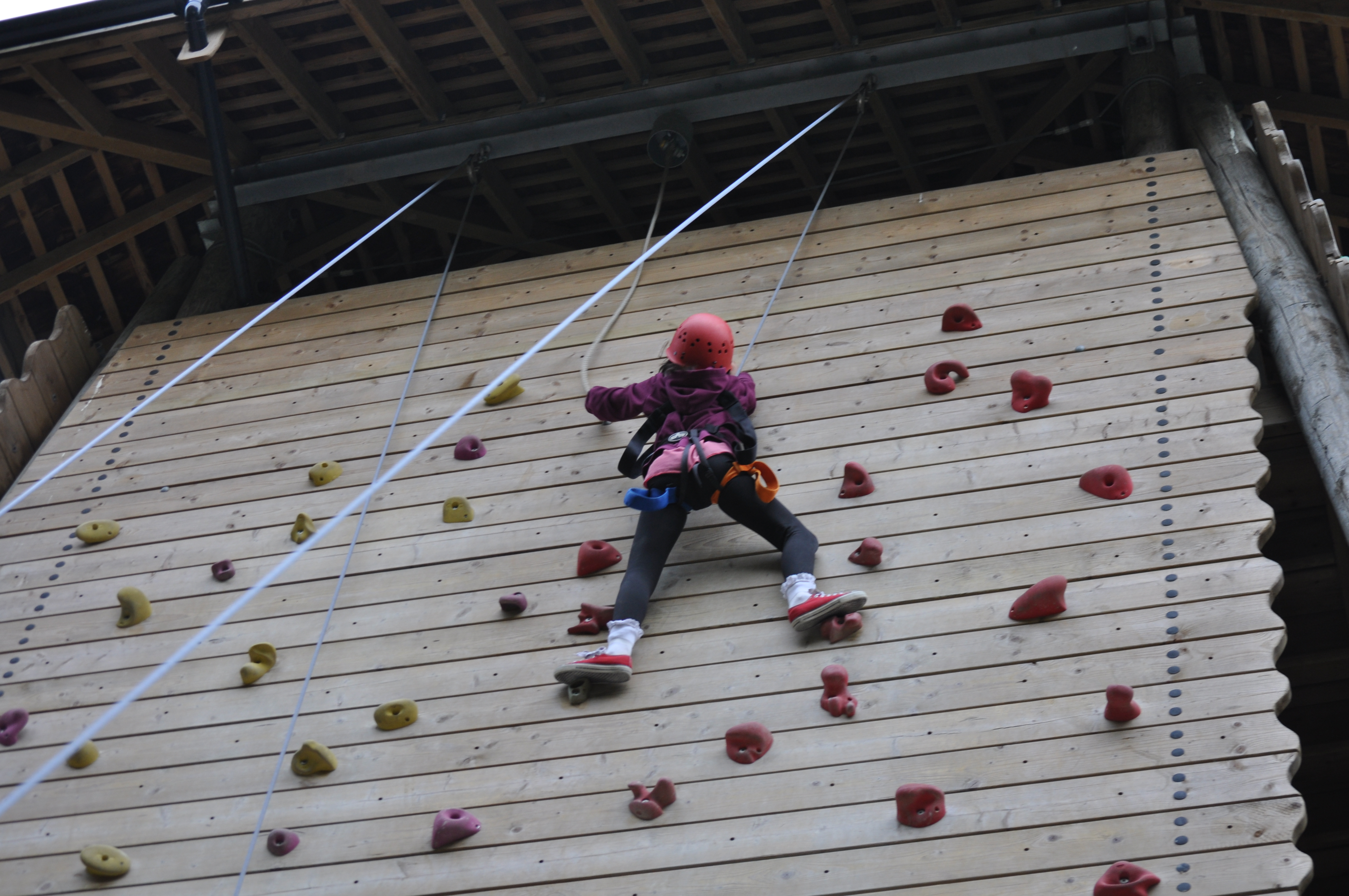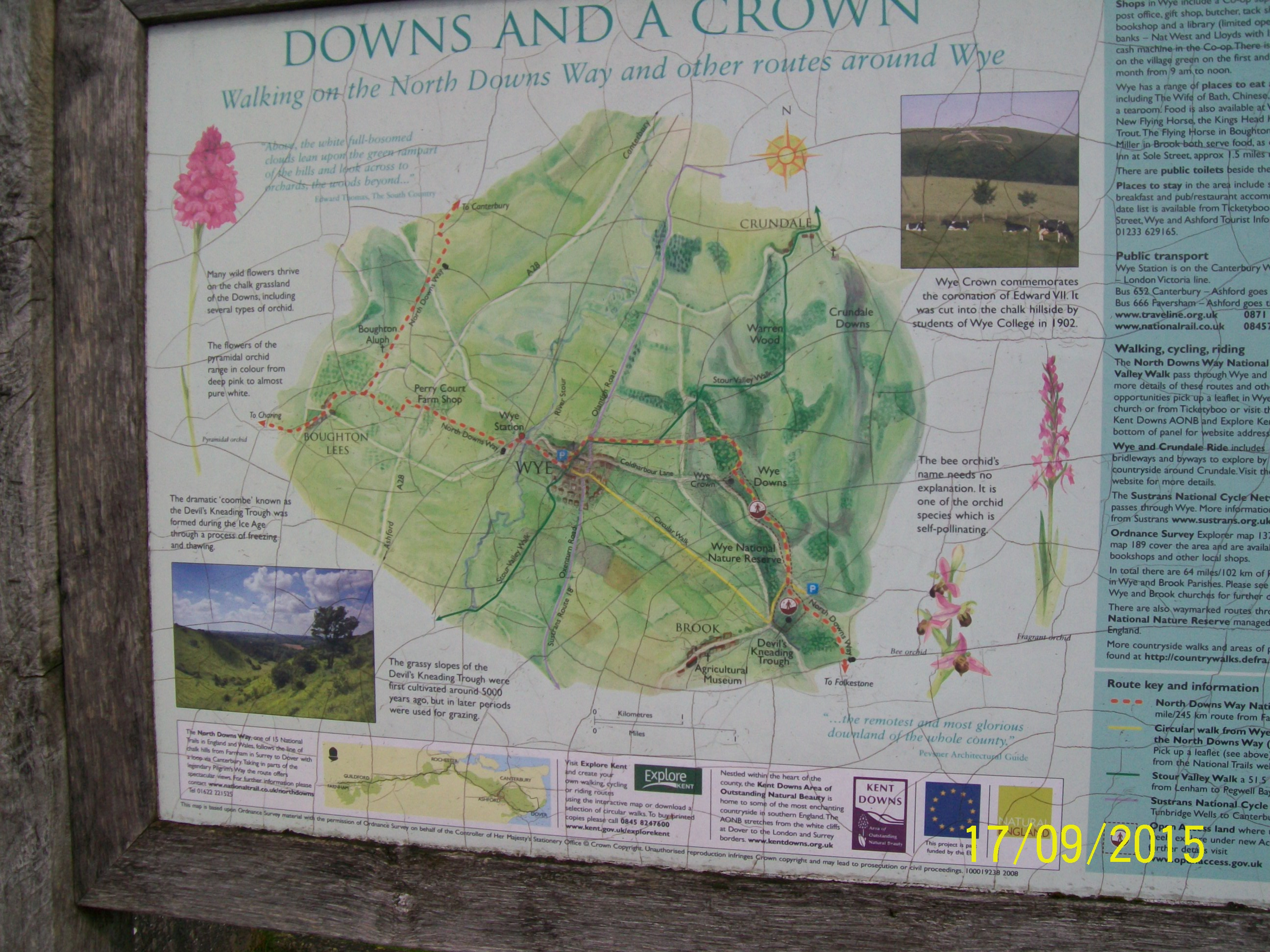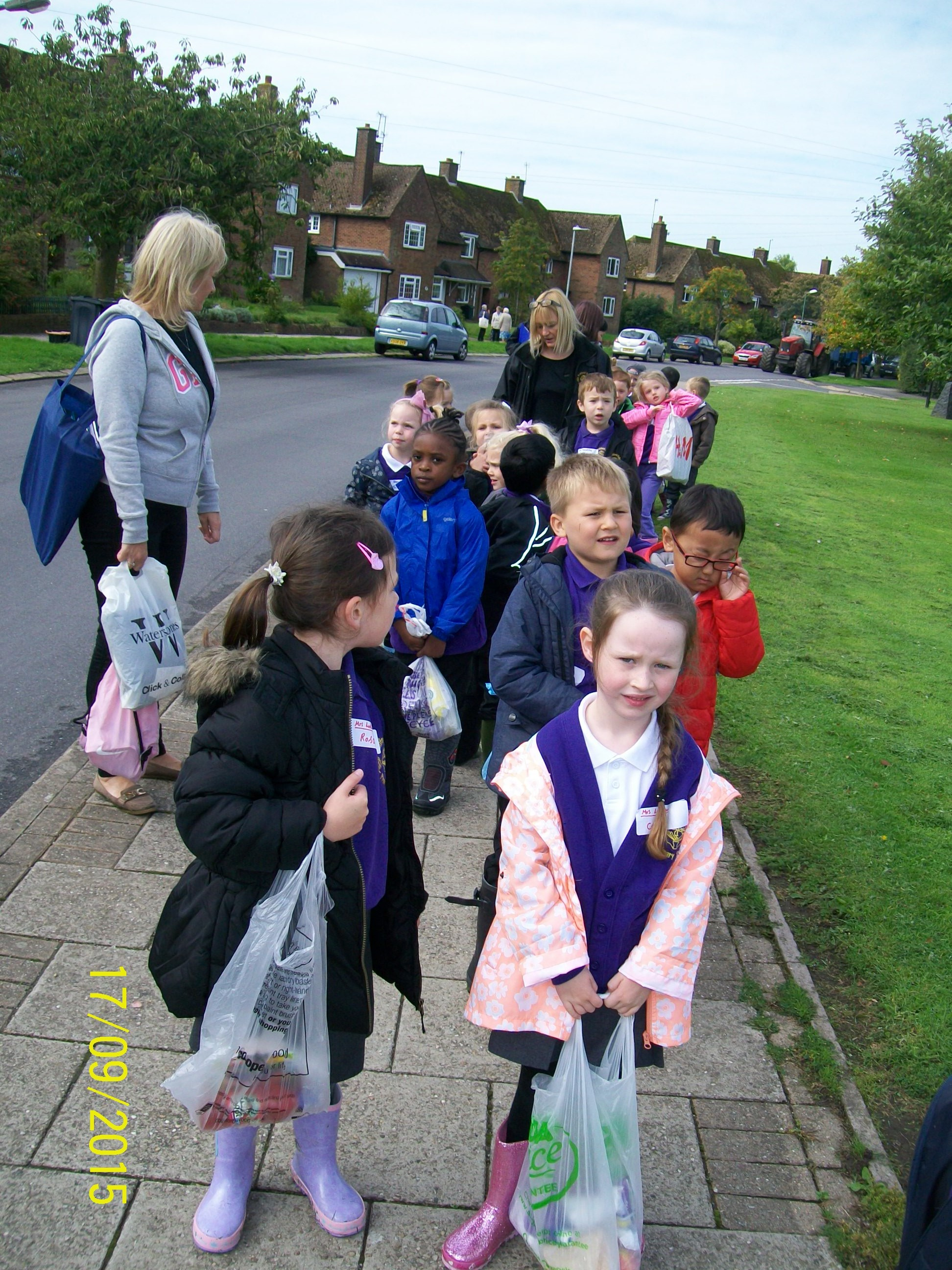Oak Tree Road  Ashford  Kent  TN23 4QR  📞01233 631259